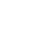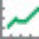# 3 projection Indicators - PBands, PO & PB

צפיות ‎7467‎
All these indicators are by Mel Widner.

Projection Bands :
-------------------------------------------------------
These project market data along the trend with the maxima and minima of the projections defining the band. The method provides a way to signal potential direction changes relative to the trend. Usage is like any other trading band.

Projection Oscillator :
-------------------------------------------------------
This indicates the relative position of price with in the bands. It fluctuates between the values 0 to 100. You can configure the "basis" to make it oscillate around a specific value (for ex., basis=50 will make it oscillate between +50 and -50). EMA of PO (length configurable, default is 5) is plotted as a signal line. There is also an option to plot the difference (oscillator - signal), just like MACD histogram. When you see a divergence in this oscillator, remember that it just indicates a potential movement with in the band (for ex., a bullish divergence shown may cause the price to cross the median and move up to the top band).

Projection Bandwidth :
-------------------------------------------------------
This shows the % width of the projection bands. A trend reversal is signaled by a high value. Low value may indicate the start of a new trend. This is also a trend strength indicator.

Borrowed the color theme for this chart from @liw0. Thanks :)
```//
// @author LazyBear
//
// If you use this code in its original/modified form, do drop me a note.
//
study("Projection Bands [LazyBear]", shorttitle="ProjectionBands_LB", overlay=true)
//length=input(14, title="Length")
length=14 // See below why this is a not an input()
sum_c=sum(cum(1),length)
psum_c=pow(sum_c,2)
sump_c=sum(pow(cum(1),2),length)
lsump_c=(length*sump_c)
denom=(lsump_c-psum_c)
rlh=((length*(sum(cum(1)*high,length)))-(sum_c*(sum(high,length))))/denom
rll=((length*(sum(cum(1)*low,length)))-(sum_c*(sum(low,length))))/denom

// Currently there is no way to do a loop. So, "length" is hardcoded to 14.
// Bands
upb=max(high,
max(high+1*rlh,
max(high+2*rlh,
max(high+3*rlh,
max(high+4*rlh,
max(high+5*rlh,
max(high+6*rlh,
max(high+7*rlh,
max(high+8*rlh,
max(high+9*rlh,
max(high+10*rlh,
max(high+11*rlh,
max(high+12*rlh,
high+13*rlh)))))))))))))

//LowerProjectionBand
lpb=min(low,
min(low+1*rll,
min(low+2*rll,
min(low+3*rll,
min(low+4*rll,
min(low+5*rll,
min(low+6*rll,
min(low+7*rll,
min(low+8*rll,
min(low+9*rll,
min(low+10*rll,
min(low+11*rll,
min(low+12*rll,
low+13*rll)))))))))))))

ul=plot(upb, linewidth=2, color=teal)
ll=plot(lpb, linewidth=2, color=teal)
plot(avg(upb,lpb), linewidth=1, color=maroon)
fill(ul,ll,color=teal)```
List of my free indicators: http://bit.ly/1LQaPK8
List of my indicators at Appstore: http://blog.tradingview.com/?p=970

## תגובותThis only shows the bands. How do you get the oscillator and bandwidth to appear?
השבDoes anyone else's Firefox crash when trying to "Make it mine"?
השבkakola
Yes, it crashed for me too. I tried with FF 29.0.1/Win7x64 HomePremium.

I use Chrome all the time, it works there perfectly.
השב